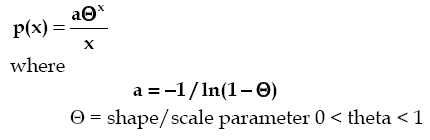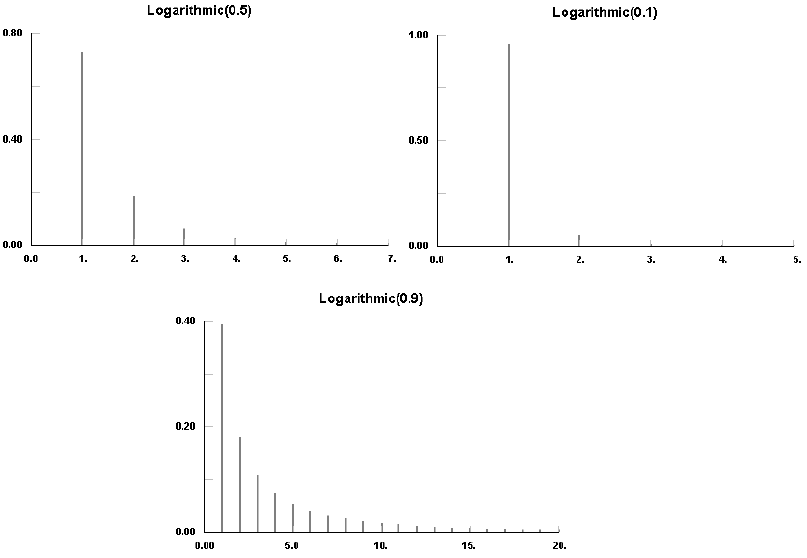AnyLogic

# logarithmicThe Logarithmic distribution is a discrete distribution bounded by [1,...]. Theta is related to the sample size and the mean.

The Logarithmic distribution is used to describe the diversity of a sample, that is, how many of a given type of thing are contained in a sample of things. For instance, this distribution has been used to describe the number of individuals of a given species in a sampling of mosquitoes, or the number of parts of a given type in a sampling of inventory. (see Johnson et.al.1).

#### Examples### logarithmic(double theta)

Description
Generates a sample of the Logarithmic distribution.
Parameters
Name Type of value Description
theta double The shape/scale parameter.
Result
Type Description
int The generated sample.

### logarithmic(double theta, java.util.Random r)

Description
Generates a sample of the Logarithmic distribution using the specified random number generator.
Parameters
Name Type of value Description
theta double The shape/scale parameter.
r java.util.Random The random number generator.
Result
Type Description
int The generated sample.

This document includes content from the “Stat::Fit User’s Manual”. Copyright 2016 Geer Mountain Software Corp.